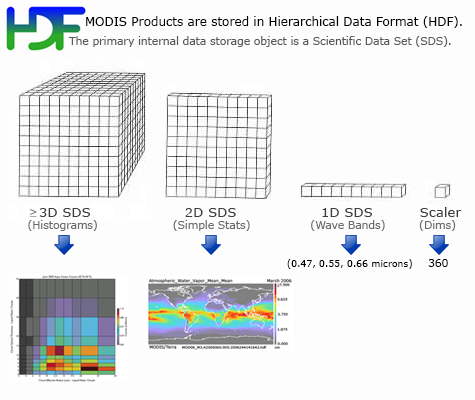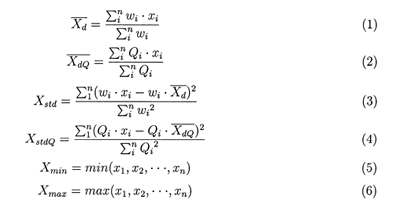Sorry, you need to enable JavaScript to visit this website.# Format & Content

Daily Product (08_D3) File Format BasicsMODIS Atmosphere Daily (08_D3) product files are stored in Hierarchical Data Format (HDF). HDF is a multi-object file format for sharing scientific data in multi-platform distributed environments. HDF files should only be accessed through HDF library subroutine and function calls, which can be downloaded from the HDF web site.

Each of the global gridded statistical fields within the HDF file is stored as a Scientific Data Set (SDS). L3 Atmosphere products contain hundreds of 1°×1° global gridded Scientific Data Sets (SDSs) or statistics derived from the L2 products of Aerosol (04_L2), Water Vapor (05_L2), Cloud (06_L2), and Atmospheric Profile (07_L2). L3 SDSs derived from Cloud Mask (35_L2) related data are actually read from (duplicated in) the Cloud (06_L2) product, therefore the L2 Cloud Mask (35_L2) product is never read (directly) into L3. The grid domain or size is 360x180 pixels, which corresponds ot 360 degrees of longitude and 180 degrees of latitude.

The SDS Inventory Table linked below shows the full set of statistics computed for each parameters -- noted by a colored circle (dot) in the table.

• Global Daily - SDS Inventory Table (Collection 6.1)   View PDF

Daily Product File (08_D3) Parameter List (primary parameters only)

Daily Product (08_D3) Statistic List

A number of statistical summaries are computed, depending on the science parameter being considered. Statistics for a given Level-2 measurement might include:

Simple Statistics

• Mean
• Minimum
• Maximum
• Standard_Deviation

QA Weighted Statistics

• QA_Mean
• QA_Standard_Deviation

Histograms of the quantity within each grid box

• Histogram_Counts

Histograms of the confidence placed in each measurement

• Confidence_Histograms

Fraction of pixels that satisfy some condition (e.g. cloudy, clear)

• Fraction

Total number of observations (pixels) within each grid box

• Pixel_Counts

Parameters of Log-Normal Distributions

• Log_Mean
• Log_Standard_Deviation

Parameters of QA Weighted Log-Normal Distributions

• QA_Log_Mean
• QA_Log_Standard_Deviation

Joint Histograms derived from comparing one science parameter to another, statistics may be computed for a subset that satisfies some condition

• Joint_Histogram_vs_Temperature
• Joint_Histogram_vs_Emissivity

Daily Product (08_D3) Statistic Computation Details

Below are more details on the computation of each statistic:

For the daily product, assume that x1, x2, ..., xn represent the retrieved pixel values of a Level-2 parameter over a 1 x 1 degree grid box, Qi is the quality flag for each retrieved pixel value, and wi is the weighting factor (1 for the daily case), then the simple statistics are defined as:In these equations, (1) will be referred to as the 'regular' mean, (2) as the QA weighted mean, (3) as the regular standard deviation, (4) as the QA weighted standard deviation, (5) as the minimum, and (6) as the maximum.

The simple statistics also include daily log regular mean, log standard deviation, log QA mean, and log QA standard deviation. These log quantities are calculated as shown in equations (1 - 4), except that x1, x2, ..., xn are replaced by their logarithms.

The histograms and joint histograms report the counts of the pixels falling into predetermined numerical intervals.

The pixel counts are used to represent the number of pixels for the parameters which do not have QA flags, while the confidence-histograms-counts are used to represent the number of counts for each parameter that falls within each QA bin (e.g., questionable, good, very good, and total).